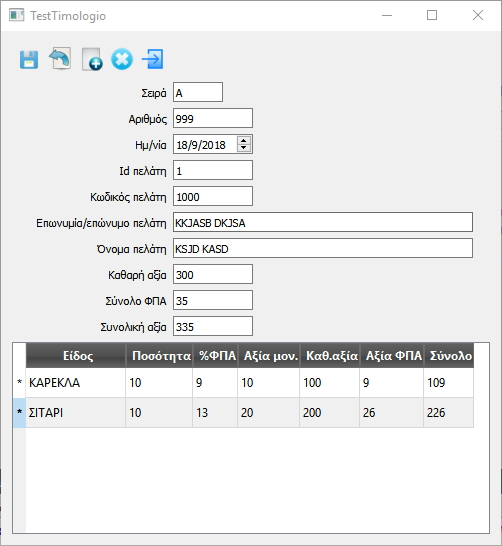How to modify QSqlTableModel's read only columns.

• Hi forum!

I have a subclass of QSqlTableModel, where I override the flags function, to make some columns read only, because I don't want the user to be able to modify them.

Qt::ItemFlags flags(const QModelIndex &index) const {
if(index.column() < 5)
return Qt::ItemIsEnabled|Qt::ItemIsSelectable;
else
return Qt::ItemIsEnabled|Qt::ItemIsSelectable|Qt::ItemIsEditable;
}

I fill my model with a table.

table_model_->setTable("some_table");
table_model_->select();

...and then I cannot modify the model data of the read only columns with setData function.

table_model_->setData(index, text)

How can I modify these data with code?

• Hi,

When are these changes happening ?

• Hi SGaist

I found that example somewhere, QStandardItemModel was the superclass at first and the setData function was able to change read only columns. I just changed the superclass to QSqlTableModel.

There is a lineedit widget connected with a slot.

connect(table_edit_, SIGNAL(textChanged(QString const&)),
this, SLOT(onEditTextChanged(QString)));

void MyTableWidget::onEditTextChanged(QString const& text)
{
foreach(QModelIndex index, table_view_->selectionModel()->selectedIndexes())
table_model_->setData(index, text);
}

• Can you show the original class code ?

• mymodel.h

#ifndef MYMODEL_H
#define MYMODEL_H

#include <QtWidgets>
#include <QtSql>

//QStandardItemModel
class MyModel : public QSqlTableModel
{
public:
MyModel(QObject* parent = 0)
: QSqlTableModel(parent)
{}

Qt::ItemFlags flags(const QModelIndex &index) const {
if(index.column() < 5)
return Qt::ItemIsEnabled|Qt::ItemIsSelectable;
else
return Qt::ItemIsEnabled|Qt::ItemIsSelectable|Qt::ItemIsEditable;
}

};

#endif // MYMODEL_H

mytablewidget.h

#ifndef MYTABLEWIDGET_H
#define MYTABLEWIDGET_H

#include "mymodel.h"
#include <QtWidgets>

class MyTableWidget : public QWidget
{
Q_OBJECT
public:
MyTableWidget(QWidget* parent = 0);

private slots:
void onEditTextChanged(QString const&);

private:
void initWidgets();
void initLayout();

QTableView* table_view_;
MyModel*    table_model_;
QLineEdit*  table_edit_;

};

#endif // MYTABLEWIDGET_H

mytablewidget.cpp

#include "mytablewidget.h"

//https://stackoverflow.com/questions/34076352/how-to-make-some-column-non-editable-and-some-column-editable-in-qtableview

MyTableWidget::MyTableWidget(QWidget* parent)
: QWidget(parent)
, table_view_(0)
, table_model_(0)
, table_edit_(0)
{
initWidgets();
initLayout();
}

void MyTableWidget::onEditTextChanged(QString const& text)
{
foreach(QModelIndex index, table_view_->selectionModel()->selectedIndexes())
table_model_->setData(index, text);
}

void MyTableWidget::initWidgets()
{
table_view_ = new QTableView(this);
table_edit_ = new QLineEdit(this);

table_model_ = new MyModel;
table_model_->setTable("timologio");
table_model_->select();
//table_model_->setColumnCount(10);
//table_model_->setRowCount(10);

//for(int c = 0; c < table_model_->columnCount(); ++c) {
//    for(int r = 0; r < table_model_->rowCount(); ++r) {
//        table_model_->setData(table_model_->index(r,c), QString("foo"));
//    }
//}

table_view_->setModel(table_model_);

connect(table_edit_, SIGNAL(textChanged(QString const&)),
this, SLOT(onEditTextChanged(QString)));

}

void MyTableWidget::initLayout()
{
QVBoxLayout* layout = new QVBoxLayout;

setLayout(layout);

}

• @Minas
I am interested in your question for my own potential future use. However I cannot test out your code. So may I aska couple of questions:

1. ...and then I cannot modify the model data of the read only columns with setData function.

What actually happens when you call setData()? Does it error/return false/do nothing, or what?

1. Why do you have an editable QLineEdit and textChanged signal for a column which is read-only?

2. Why/where are you wanting to change the data in a read-only column?

• Hi Jon

1. setData returns false.

2,3. The code is just an example. The real case is an Invoice, where in the QTableView the user inserts items.
For each line, the user selects the item from a combo and types the quantity.
There must be other columns where the system has to calculate for that quantity, the price, the VAT & the total price, based on data of another table.
Of course the user must not be able to edit these columns!

Like this pic:• The user selects column #1
The system updates column#3 and column#4 from another table
The user types the quantity in column#2
The system calculates and updates the rest column#5, column#6 & column#7.

• @Minas
Thanks for the clarification on setData()!

To be clear: I am not fluent in Greek, so I don't know what is what on your dialog! Are you only connecting the textChanged signal to those QLineEdits which correspond to columns the user is allowed to edit? For those which are calculated read-only, in addition to clearing the Qt::ItemIsEditable flag in the model you are either not showing them in your QLineEdits above the table or the line edit is itself read-only, yes?

Given that nearly all the columns seem to be calculated, why are you using a QSqlTableModel and not a QSqlQueryModel, just so that I know?

• I repeat this is an example!

eidhModel is the model of the QTableView.
updatePosa is the function that calculates the prices etc.

So I make the connection:

connect(eidhModel,SIGNAL(dataChanged(QModelIndex,QModelIndex)),this,SLOT(updatePosa(QModelIndex,QModelIndex)));

and the function is:

void MainWindow::updatePosa(QModelIndex tl,QModelIndex br)
{
Q_UNUSED(br)

if (tl.column() != 2 && tl.column() != 3) //Είδος or Ποσότητα
return;

if (tl.column() == 2) //Είδος
{
QVariant eidosId = eidhModel->record(tl.row()).field(tl.column()).value();
QSqlQuery q;
//Βρίσκω την τιμή μονάδος
q.exec(tr("select * from eidos where id = %1").arg(eidosId.toInt()));
if (q.next())
{
QVariant axiaMon = q.value(4);
eidhModel->setData(eidhModel->index(tl.row(), 5), axiaMon);
//Βρίσκω το ποσοστό ΦΠΑ
QVariant fpaId = q.value(3);
q.exec(tr("select * from fpa where id = %1").arg(fpaId.toInt()));
if (q.next())
{
QVariant fpaPc = q.value(2);
eidhModel->setData(eidhModel->index(tl.row(), 4), fpaPc);
}
}
}
QVariant posotita = eidhModel->record(tl.row()).field(3).value();
QVariant axiaMon = eidhModel->record(tl.row()).field(5).value();
QVariant fpaPc = eidhModel->record(tl.row()).field(4).value();

double axiaKath = posotita.toDouble() * axiaMon.toDouble();
eidhModel->setData(eidhModel->index(tl.row(), 6), axiaKath);

double axiaFpa = axiaKath * fpaPc.toDouble() * 0.01;
eidhModel->setData(eidhModel->index(tl.row(), 7), axiaFpa);

double axiaSyn = axiaKath + axiaFpa;
eidhModel->setData(eidhModel->index(tl.row(), 8), axiaSyn);

}

• Anyway, the fact that the function setData does not update columns where Qt::ItemIsEditable is not set, is normal for Qt?

• @Minas
I guess so, since you say that is the behaviour!

Which seems to leave you with two possible choices:

• Add in Qt::ItemIsEditable just before you want to setData() from back-end, and restore flags immediately afterward!

• Assuming it works/can be done, instead of Qt::ItemIsEditableuse doc.qt.io/qt-5/qitemdelegate.html to call the base class implementation for all columns which are editable and "do nothing" for those which are not?

• OK the (obvious!!!) solution is to remove Qt::ItemIsEnabled and not the Qt::ItemIsEditable in columns I don't want the user to edit. The only disadvantage is that the user cannot select cells on these columns.

Thank you all!!!

Minas